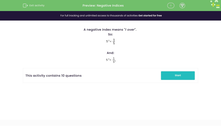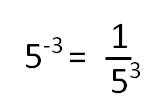# Negative Indices

In this worksheet, students understand and use negative indices.Key stage:  KS 3

Curriculum topic:   Number

Curriculum subtopic:   Understand Integer Powers/Real Roots

Difficulty level:#### Worksheet Overview

What is a negative indices? A number or term with a negative index means it can be written as a reciprocal of that number.

For example,And:How do we get negative indices?

Negative indices occur when multiplying or dividing two terms where the the result of adding or subtracting the powers gives a negative value.

For example,

a3 × a-5 = a3 + - 5 = a-2 = 1/a2

or

p1 ÷ p2 = p1-2 = p-1= 1/p

### What is EdPlace?

We're your National Curriculum aligned online education content provider helping each child succeed in English, maths and science from year 1 to GCSE. With an EdPlace account you’ll be able to track and measure progress, helping each child achieve their best. We build confidence and attainment by personalising each child’s learning at a level that suits them.

Get started